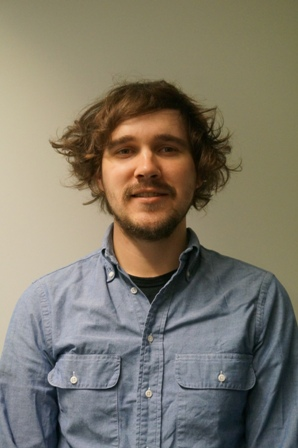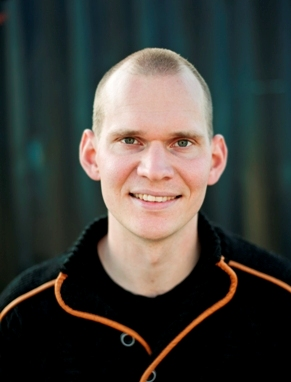# Percolation and Structural Graph Parameters

On 30 January – 3 February 2017 NETWORKS organizes the fourth Training Week for PhD Students of NETWORKS. The topics of this Training Week are a minicourse on percolation and one on structural graph parameters. Tim Hulshof and Bart Jansen are the main lecturers in the morning during this Training Week.

In the afternoon various Networks PhD students and other Networks researchers will give talks about their recent results. There will also be time for joint research in small groups (either in existing collaborations or in new collaborations) in so-called working sessions.

## A minicourse on percolation

This lecture is given by Tim Hulshof.In this minicourse we will discuss some of the basics of percolation theory.

Percolation is a paradigm model in probability theory (and physics). It is simply defined as follows: Take a graph and remove from it at random a fraction of the edges (or vertices). We call the remainder the percolation of the graph.

It does not take a powerful imagination to see that this model has plenty of useful applications. But besides that, percolation is also a topic of great mathematical riches. This explains why percolation has been one of the main topics in modern probability for over 70 years now.

Some typical questions percolation theorists try to answer are:

• Is the percolation connected?
• Does the percolation have an infinite component? (Assuming we started with an infinite graph.)
• How does the structure of the percolation change when we change the fraction of removed edges?
• How does the geometry of the graph influence the geometry of the percolation?
• How does random walk on the percolation behave?

It turns out that each of these questions has a number of interesting answers.

The course will have the following (highly tentative) schedule:

1. The main results and important applications of percolation theory.
2. Correlation inequalities and other useful tools.
3. Universality classes.
4. The ant in the labyrinth: random walk on percolation clusters.

The course will be largely based on the Book “Percolation”, by Geoffrey Grimmett.

## Minicourse on Structural graph parameters

This lecture is given by Bart Jansen.This minicourse deals with structural graph parameters and the relations between them. A graph parameter assigns a number to a graph, by answering a question such as ‘how many edges are there?’, `how many vertices are needed to intersect all the cycles?’, or ‘what is the size of the largest clique?’.

Over the last decades, the study of structural graph theory has produced a rich toolbox of different graph parameters that also capture more subtle aspects of the structure of a graph. For example, the parameter treewidth gives a formal way to answer the question: ‘how treelike is this graph?’.

The goal of the minicourse is to introduce useful graph parameters and relate them to each other. The hierarchy among graph parameters facilitates a research methodology that has proven very useful: start by exploring the research question of interest on graphs that are structurally simple according to a `very restrictive’ parameter, and repeatedly generalize the resulting insights to more and more general parameters.

The concepts of structural graph theory also find applications in parameterized computational complexity. When faced with a computational problem that is intractable (NP-complete) in general, one can study whether it can be solved efficiently on large graphs that are structurally simple in terms of a chosen parameter. Investigating how the computational complexity of the problem depends on the value of a structural parameter results in an extended dialogue with a computational problem that yields important insights in the types of inputs for which it can be solved efficiently.

If time permits, we will explore some examples of the application of structural graph theory to parameterized complexity.

## Location

Conferentiecentrum Kaap Doorn , Postweg 9, 3941 KA   Doorn,  http://www.kaapdoorn.com/